# 一篇文章让你学会绘画十一种常见数据分析图（折线图，直方图，散点图，雷达图等等）1. 折线图

plt.plot绘制折线图代码展示：

2. 散点图

scatter绘图示例1：

3. 直方图

bar绘图示例1：

bar绘图示例2：

4. 饼图

pie绘图示例：

5. 箱线图

boxplot绘图示例：

6.概率图

7.雷达图

8.流向图

9.绘图中的表格设置

10. 极坐标图

11. 词云图

11.1 安装相关的包

11.2 词云生成过程

11.3 词云生成示例：

## 1. 折线图

matplotlib.pyplot.plot(*args,**kwargs)

plot函数在官方文档的语法中只要求填入不定长参数，实际可以填入的主要参数及其说明见下表1

color参数的8种常用的缩写见表2

 颜色缩写 代表的颜色 b 蓝色 g 绿色 r 红色 c 青色 m 品红 y 黄色 k 黑色 w 白色

### plt.plot绘制折线图代码展示：

import matplotlib.pyplot as plt
import numpy as np
%matplotlib inline
x = np.arange(9)
y = np.sin(x)
z = np.cos(x)
#marker数据点样式，linewidth线宽，linestyle线型样式，color颜色
plt.plot(x,y,marker='*',linewidth=1,linestyle='--',color='orange')
plt.plot(x,z)
plt.title('matplotlib AK')
plt.xlabel('height',fontsize=15)
plt.ylabel('width',fontsize=15)
#设置图例
plt.legend(['Y','Z'],loc='upper right')
plt.grid(True)
plt.show()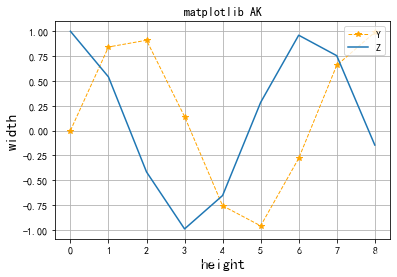## 2. 散点图

scatter方法的格式：

        matplotlib.pyplot.scatter(x,y,s=None,c=None,marker=None,alpha=None)

scatter函数主要参数及其说明见表3

 参数 说明 x,y 接收array，表示X轴和Y轴对应的数据，无默认 s 接收数值或一维的array，指定点的大小，若传入一维array则表示每个点的大小，默认为None c 接收颜色或一维的array，指定点的颜色，若传入一维array则表示每个点的颜色，默认为None marker 接收特定的string，表示绘制的点的类型，默认为None alpha 接收0~1的小数，表示点的透明度，默认为None

### scatter绘图示例1：

fig.ax = plt.subplots()
plt.rcParams['font.family'] = ['SimHei']      #用来显示中文标签
plt.rcParams['axes.unicode_minus'] = False   #用来正常显示符号
x1 = np.arange(1,30)
y1 = np.sin(x1)
ax1 = plt.subplot(1,1,1)
plt.title('散点图 AK')
plt.xlabel('X')
plt.ylabel('Y')
lvalue = x1
ax1.scatter(x1,y1,c='r',s=100,linewidths=lvalue,marker='o')
plt.legend('x1')
plt.show()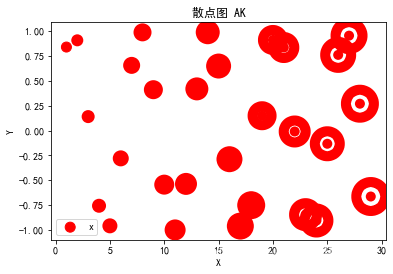scatter绘图示例2：

fig,ax = plt.subplots()
plt.rcParams['font.family'] = ['SimHei']      #用来显示中文标签
plt.rcParams['axes.unicode_minus'] = False   #用来正常显示符号
for color in ['red','green','blue']:
n = 500
x,y = np.random.randn(2,n)
ax.scatter(x,y,c=color,label=color,alpha=0.3,edgecolor='none')
ax.legend()
ax.grid(True)
plt.show()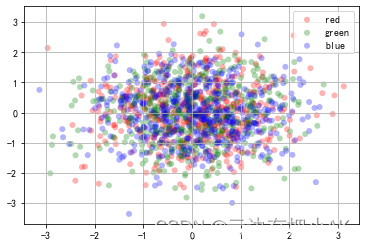## 3. 直方图

matplotlib.pyplot.bar(left,height,width = 0.8,bottom = None,hold = None,data = None)


 参数 说明 left 接收array，表示X轴数据，无默认 height 接收array，表示X轴所代表数据的数量，无默认 width 接收0~1的float，指定直方图宽度，默认为0.8 color 接收特定string或者包含颜色字符串的array，表示直方图颜色，默认为None

### bar绘图示例1：

import pandas as pd
import matplotlib.pyplot as plt
import numpy as np
fig,axes = plt.subplots(2,1)
data = pd.Series(np.random.randn(16),index=list('abcdefghijklmnop'))
data.plot.bar(ax = axes,color='k',alpha=0.7)
data.plot.barh(ax = axes,color='k',alpha=0.7)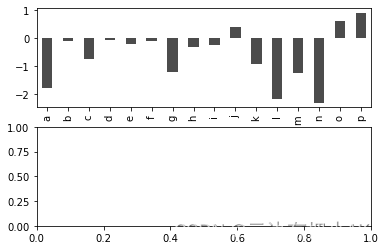### bar绘图示例2：

import pandas as pd
import matplotlib.pyplot as plt
import numpy as np
plt.rcParams['font.family'] = ['SimHei']      #用来显示中文标签
plt.rcParams['axes.unicode_minus'] = False   #用来正常显示符号
fig,ax = plt.subplots()
x = np.arange(1,6)
y1 = np.random.uniform(1.5,1.0,5)
y2 = np.random.uniform(1.5,1.0,5)
plt.bar(x,y1,width = 0.35,facecolor='lightskyblue',edgecolor = 'white')
plt.bar(x+0.35,y2,width = 0.35,facecolor='yellowgreen',edgecolor = 'white')
plt.show()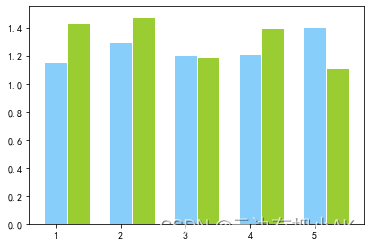## 4. 饼图

matplotlib.pyplot.pie(x,explode = None,labels = None,color = None,autopct = None,
pctdistance = 0.6,shadow=false,labeldistance=1.1,startangle=None,radius=None,...)

pie函数常用参数及其说明见表5

 参数 说明 x 接收array，表示用于绘制饼图的数据，无默认 explode 接收array，指定项离饼图圆心为n个半径，默认为None labels 接收array，指定每一项的名称，默认为None color 接收特定的string或包含颜色字符串的array，表示颜色，默认为None autopct 接收特定的string，指定数值的显示方式，默认为None pctdistance float型，指定每一项的比例和距离饼图圆心n个半径，默认为0.6 labeldistance float型，指定每一项的名称和距离饼图圆心的半径数，默认为1.1 radius float型，表示饼图的半径，默认为1

### pie绘图示例：

plt.figure(figsize=(6,6))
#建立轴的大小
labels = ['Springs','Summer','Autumn','Winter']
x = [15,30,45,10]
explode = (0.05,0.05,0.05,0.05)
#这个是控制分离的距离的，默认饼图不分离
plt.pie(x,labels = labels,explode = explode,startangle = 60,autopct='%1.1f%%')
#autopct在图中显示比例值，注意值的格式
plt.title('Rany days by season')
plt.show()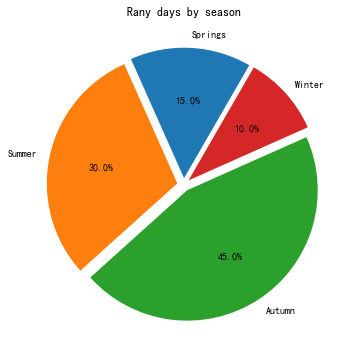## 5. 箱线图

boxplot函数的格式：

matplotlib.pyplot.boxplot(x,notch = None,sym = None,vert = None,whis = None,
positions = None,width = None,patch_artist = None,
meanline = None,labels = None,...)



boxplot函数常用参数及其说明见表6：

 参数 说明 x 接收array，表示用于绘制箱线图的数据，无默认 notch 接收boolean，表示中间箱体是否有缺口，默认为None sym 接收特定的string，指定异常点形状，默认为None vert 接收boolean，表示图形是纵向的或者横向，默认为None positions 接收array，表示图形位置，默认为None width 接收scalar或者array，表示每个箱体的宽度，默认为None labels 接收array，指定每一个箱线图的标签，默认为None meanline 接收boolean，表示是否显示均值线，默认为False

### boxplot绘图示例：

import numpy as np
import pandas as pd
import matplotlib.pyplot as plt
np.random.seed(2)#设置随机种子
df = pd.DataFrame(np.random.rand(5,4),columns = ['A','B','C','D'])
#生成0~1的5*4维度数据并存入4列DataFrame中
df.boxplot()
plt.show()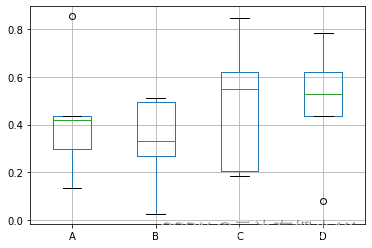## 6.概率图

### 绘制概率图：

import numpy as np
import pandas as pd
import matplotlib.pyplot as plt
from scipy.stats import norm
fig,ax = plt.subplots()
plt.rcParams['font.family'] = ['SimHei']      #用来显示中文标签
plt.rcParams['axes.unicode_minus'] = False   #用来正常显示符号
np.random.seed(1587554)
mu = 100
sigma = 15
x = mu+sigma*np.random.randn(437)
num_bins = 50
n,bins,patches = ax.hist(x,num_bins,density=1,stacked=True)
y = norm.pdf(bins,mu,sigma)
ax.plot(bins,y,'--')
fig.tight_layout()
plt.show()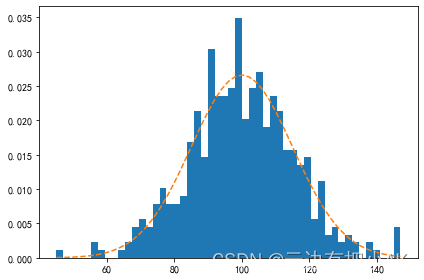## 7.雷达图

### 绘制某学生成绩信息的雷达图

import matplotlib.pyplot as plt
import numpy as np
%matplotlib inline
#某学生的课程与成绩
courses = ['数据结构','数据可视化','高数','英语','软件工程','组成原理','C语言','体育']
scores = [82,95,78,85,45,88,76,88]
dataLength = len(scores) #数据长度
#angles数组把圆周等分为dataLength份
angles = np.linspace(0,2*np.pi,dataLength,endpoint=False)
scores.append(scores)
angless = np.append(angles,angles)  #闭合
#绘制雷达图
plt.polar(angless,   #设置角度
scores,   #设置各个角度上的数据
'rv--',   #设置颜色、线型和端点符号
linewidth=2)  #设置线宽
#设置角度网络标签
plt.thetagrids(angles*180/np.pi,courses,fontproperties='simhei',fontsize=12,color='k')
#填充雷达图内部
plt.fill(angless,scores,facecolor='g',alpha=0.2)
plt.show()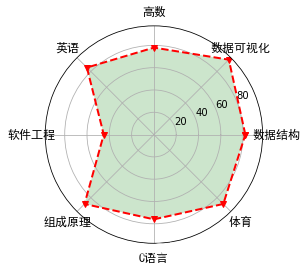## 8.流向图

### 流向图绘制

import numpy as np
import matplotlib.pyplot as plt
Y,X = np.mgrid[-3:3:100j,-3:3:100j]
U = -1-X**2+Y
V = 1+X-Y**2
speed = np.sqrt(U*U+V*V)
plt.streamplot(X,Y,U,V,color=U,linewidth = 2,cmap = plt.cm.autumn)
plt.colorbar()
f,(ax1,ax2) = plt.subplots(ncols=2)
ax1.streamplot(X,Y,U,V,density=[0.5,1])
lw = 5*speed/speed.max()
ax2.streamplot(X,Y,U,V,density=0.6,color='k',linewidth=lw)
plt.show()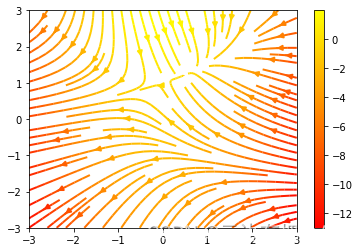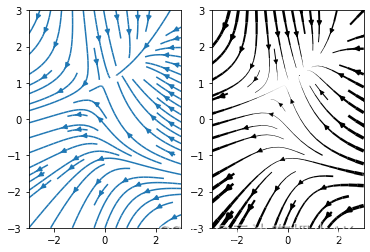## 9.绘图中的表格设置

### 在绘图中显示数据表格

import numpy as np
import matplotlib.pyplot as plt
plt.rcParams['font.family'] = ['SimHei']
data = [[66386,174296,75131,577908,32015],
[58230,381139,78045,99308,160454],
[89135,80552,152558,497981,603535],
[78415,81858,150656,193263,69638],
[139361,331509,343164,781380,52269]]
columns = ('Freeze','Wind','Flood','Quake','Hail')
rows = ['%d year'% x for x in (100,50,20,10,5)]
values = np.arange(0,2500,500)
value_increment = 1000
colors = plt.cm.BuPu(np.linspace(0,0.5,len(columns)))
n_rows=len(data)
index = np.arange(len(columns))+0.3
bar_width=0.4
y_offset = np.array([0.0]*len(columns))
cell_text = []
for row in range(n_rows):
plt.bar(index,data[row],bar_width,bottom=y_offset)
y_offset = y_offset+data[row]
cell_text.append(['%1.1f'%(x/1000.0) for x in y_offset])
colors = colors[::-1]
cell_text.reverse()
the_table = plt.table(cellText=cell_text,
rowLabels=rows,
rowColours = colors,
colLabels = columns,
loc = 'bottom')
plt.ylabel("Loss in ${0}'s".format(value_increment)) plt.yticks(values*value_increment,['%d' % val for val in values]) plt.xticks([]) plt.title('气象灾害损失') plt.show()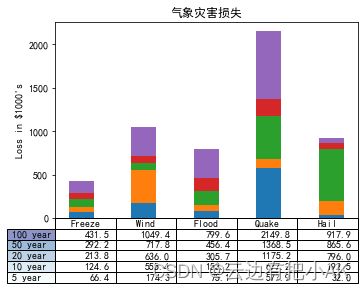## 10. 极坐标图 在平面投影中，由X轴和Y轴定位坐标；而在极坐标投影中，需要以半径和角度的形式定位坐标。极坐标投影中的半径以圆半径的大小显示，并且以每个角度为0°的圆的角度为起点投影角度。要生成极坐标投影，需要将投影类型定义为极坐标。 ### 绘制极坐标图 import numpy as np import matplotlib.pyplot as plt r = np.linspace(0,2,100) theta = 2*np.pi*r fig = plt.figure(figsize=(13,4)) ax1 = plt.subplot(121,projection='polar') ax1.scatter(theta,r,label='Polar Projection',s=10) ax1.legend(bbox_to_anchor=(0.85,1.35)) ax2 = plt.subplot(122) ax2.scatter(theta,r,label='Polar Projection',s=10) ax2.legend(bbox_to_anchor=(0.85,1.35)) ax2.set_xlabel('R') ax2.set_ylabel(r'$\theta\$')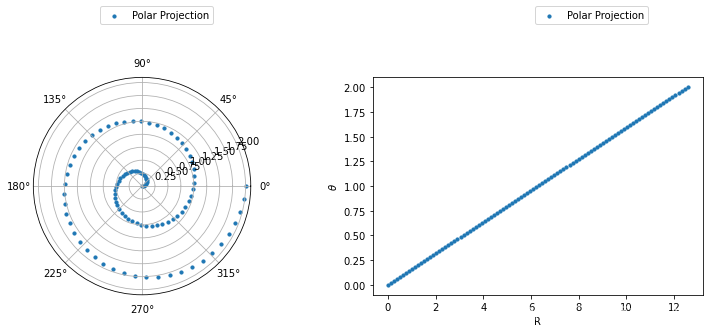## 11. 词云图

### 11.1 安装相关的包

pip install wordcloud
pip install jieba

### 11.2 词云生成过程

1）使用Pandas读取数据并将需要分析的数据转化为列表
2）对获得的列表数据使用分词工具jieba进行遍历分词

3）使用WordCloud设置词云图片的属性、掩码和停用词，并生成词云图像。

### 11.3 词云生成示例：

https://blog.csdn.net/weixin_52797843/article/details/122547594?spm=1001.2014.3001.5502

## 结尾：